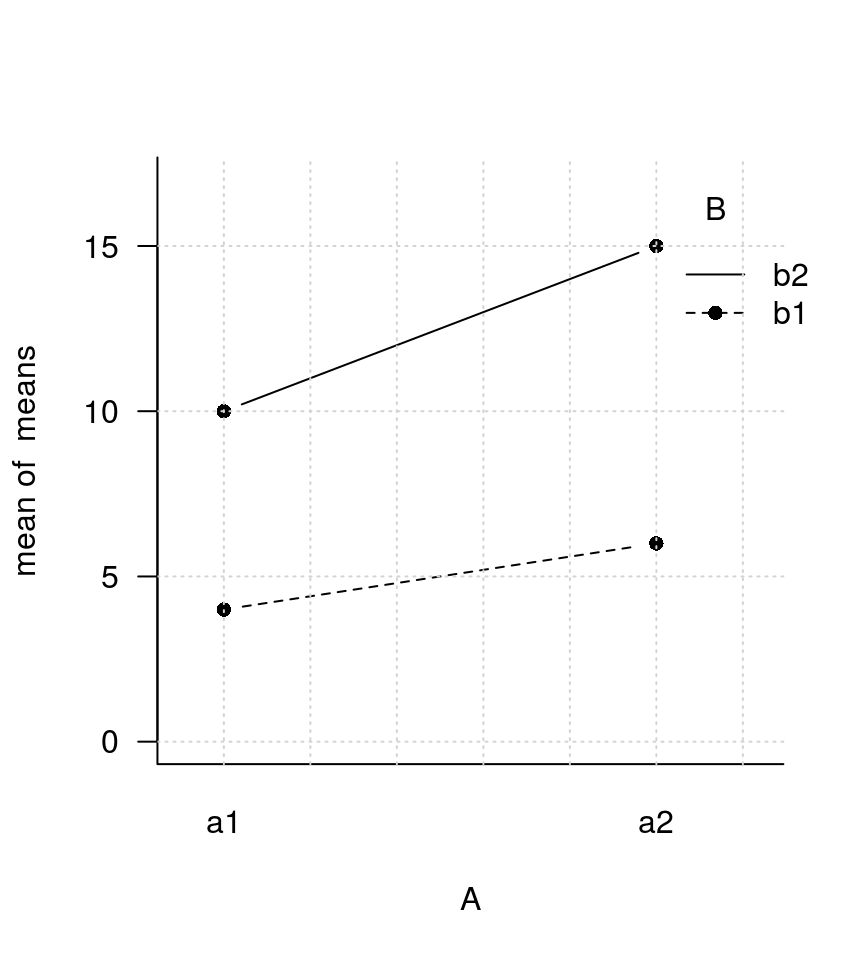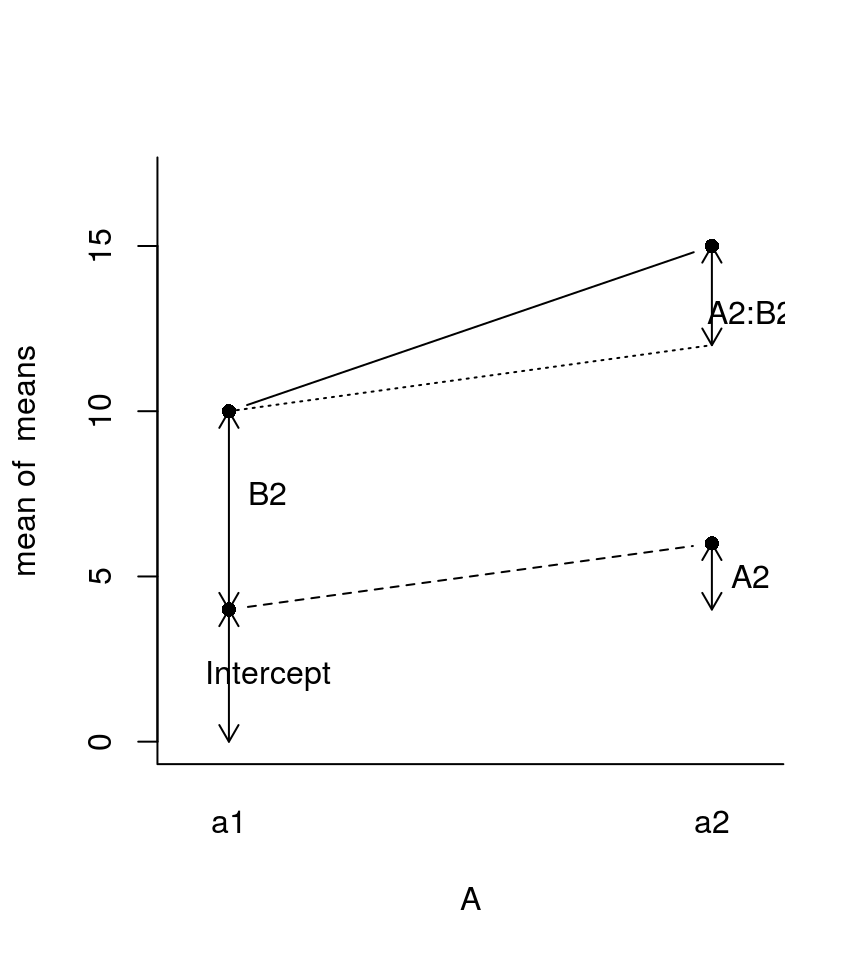Everyone is welcome here --- except those who have borrowed books from me for and have not returned them yet!

# Interpreting the coefficients of linear models in a 2x2 design

Posted on août 25, 2012 in stats

# Creating a 2x2 factorial design

Let us create a 2x2 design, resulting from the crossing of two binary factors “A” and “B”:

A <- gl(2, 1, 4, labels=c('a1', 'a2'))
B <- gl(2, 2, 4, labels=c('b1', 'b2'))
means <- c(4, 6, 10, 15)
tapply(means, list(A=A, B=B), mean)
##     B
## A    b1 b2
##   a1  4 10
##   a2  6 15
par(las=1)
interaction.plot(A, B, means,
ylim=c(0, 17),
legend=T,
type='b',
pch=16,
bty="l")
grid()To obtain the main effects of A and B:

(Ameans <- tapply(means, list(A), mean))
##   a1   a2
##  7.0 10.5
diff(Ameans)  # main effect of A
##  a2
## 3.5
(Bmeans <- tapply(means, list(B), mean))
##   b1   b2
##  5.0 12.5
diff(Bmeans)  # main effect of B
##  b2
## 7.5

### treatment coding (contr.treat)

Let us fit an additive model using the formula “A * B”:

summary(modadd <- lm(means ~ A + B))
##
## Call:
## lm(formula = means ~ A + B)
##
## Residuals:
##     1     2     3     4
##  0.75 -0.75 -0.75  0.75
##
## Coefficients:
##             Estimate Std. Error t value Pr(>|t|)
## (Intercept)    3.250      1.299   2.502    0.242
## Aa2            3.500      1.500   2.333    0.258
## Bb2            7.500      1.500   5.000    0.126
##
## Residual standard error: 1.5 on 1 degrees of freedom
## Multiple R-squared:  0.9682, Adjusted R-squared:  0.9046
## F-statistic: 15.22 on 2 and 1 DF,  p-value: 0.1783

Notice that the estimates of the coefficients labeled Aa2 and Bb2 correspond to the main effects of A (3.50) and B (7.50). This is because, in R, factors use by default a contr.treatment parametrization. You can see that using the contrasts function and by displaying the matrix of the linear model:

getOption('contrasts')
##         unordered           ordered
## "contr.treatment"      "contr.poly"
contrasts(A)
##    a2
## a1  0
## a2  1
contrasts(B)
##    b2
## b1  0
## b2  1
model.matrix(modadd)
##   (Intercept) Aa2 Bb2
## 1           1   0   0
## 2           1   1   0
## 3           1   0   1
## 4           1   1   1
## attr(,"assign")
##  0 1 2
## attr(,"contrasts")
## attr(,"contrasts")$A ##  "contr.treatment" ## ## attr(,"contrasts")$B
##  "contr.treatment"

An observation can belong to one of the four classes a1b1, a1b2, a1b2 or a2b2. The class can be indicated by a coding vector, as shown below.

class   coding vector   expected_value
a1b1       1 0 0           intercept
a2b1       1 1 0           intercept + a2
a1b2       1 0 1           intercept + b2
a2b2       1 1 1           intercept + a2 + b2

The cross-product of the coding vector by the estimated coefficients yields the expected value given the class.

### deviation coding (contr.sum)

It is possible to use a different coding scheme (aka “parametrization”), for example “deviation coding” using contr.sum:

contr.sum(2)
##   [,1]
## 1    1
## 2   -1
contrasts(A) <- contr.sum(2)
contrasts(B) <- contr.sum(2)

modadd2 <- lm(means ~ A + B)
model.matrix(modadd2)
##   (Intercept) A1 B1
## 1           1  1  1
## 2           1 -1  1
## 3           1  1 -1
## 4           1 -1 -1
## attr(,"assign")
##  0 1 2
## attr(,"contrasts")
## attr(,"contrasts")$A ## [,1] ## a1 1 ## a2 -1 ## ## attr(,"contrasts")$B
##    [,1]
## b1    1
## b2   -1
summary(modadd2)
##
## Call:
## lm(formula = means ~ A + B)
##
## Residuals:
##     1     2     3     4
##  0.75 -0.75 -0.75  0.75
##
## Coefficients:
##             Estimate Std. Error t value Pr(>|t|)
## (Intercept)     8.75       0.75  11.667   0.0544 .
## A1             -1.75       0.75  -2.333   0.2578
## B1             -3.75       0.75  -5.000   0.1257
## ---
## Signif. codes:  0 '***' 0.001 '**' 0.01 '*' 0.05 '.' 0.1 ' ' 1
##
## Residual standard error: 1.5 on 1 degrees of freedom
## Multiple R-squared:  0.9682, Adjusted R-squared:  0.9046
## F-statistic: 15.22 on 2 and 1 DF,  p-value: 0.1783

The coefficients now represent the distance to the global mean for a1 and b1.

Note: The aov function also allows to display effect sizes, as deviations from the global average.

model.tables(aov(means ~ A + B))
## Tables of effects
##
##  A
## A
##    a1    a2
## -1.75  1.75
##
##  B
## B
##    b1    b2
## -3.75  3.75

## Model with interaction

### treatment coding

Next, let us fit a new model that includes the interaction term in addition to the main effects. To this end, we use the formula “A * B” as a shorhand to “A + B + A:B”:

contrasts(A) <- contr.treatment  # setting the parametrization
contrasts(B) <- contr.treatment  # back to the default

mod1 = lm(means ~ A * B)
summary(mod1)
##
## Call:
## lm(formula = means ~ A * B)
##
## Residuals:
## ALL 4 residuals are 0: no residual degrees of freedom!
##
## Coefficients:
##             Estimate Std. Error t value Pr(>|t|)
## (Intercept)        4         NA      NA       NA
## A2                 2         NA      NA       NA
## B2                 6         NA      NA       NA
## A2:B2              3         NA      NA       NA
##
## Residual standard error: NaN on 0 degrees of freedom
## Multiple R-squared:      1,  Adjusted R-squared:    NaN
## F-statistic:   NaN on 3 and 0 DF,  p-value: NA

(Remark that because the model fits perfectly the data, all inferential stats are NA)

The following plot shows the interpretation of the coefficients.

interaction.plot(A, B, means,
ylim=c(0, 17),
legend=F,
type='b',
pch=16,
bty="l")

eps <- 0.9

lines(c(1, 2), c(10, 12), lty=3)

arrows(1, 0, 1, 4, code=3, length=.1)
arrows(2, 4, 2, 6, code=3, length=.1)
arrows(1, 4, 1, 10, code=3, length=.1)
arrows(2, 12, 2, 15, code=3, length=.1)

text(1.08, 2, "Intercept")
text(2.08, 5, "A2")
text(1.08, 7.5, "B2")
text(2.08, 13, "A2:B2")model.matrix(mod1)
##   (Intercept) A2 B2 A2:B2
## 1           1  0  0     0
## 2           1  1  0     0
## 3           1  0  1     0
## 4           1  1  1     1
## attr(,"assign")
##  0 1 2 3
## attr(,"contrasts")
## attr(,"contrasts")$A ## 2 ## a1 0 ## a2 1 ## ## attr(,"contrasts")$B
##    2
## b1 0
## b2 1
model.tables(aov(means ~ A * B))
## Tables of effects
##
##  A
## A
##    a1    a2
## -1.75  1.75
##
##  B
## B
##    b1    b2
## -3.75  3.75
##
##  A:B
##     B
## A    b1    b2
##   a1  0.75 -0.75
##   a2 -0.75  0.75

### deviation coding

Let us now see what happens when we change the parametrization to deviation coding.

contrasts(A) <- contr.sum
contrasts(B) <- contr.sum

mod2 <- lm(means ~ A * B)
model.matrix(mod2)
##   (Intercept) A1 B1 A1:B1
## 1           1  1  1     1
## 2           1 -1  1    -1
## 3           1  1 -1    -1
## 4           1 -1 -1     1
## attr(,"assign")
##  0 1 2 3
## attr(,"contrasts")
## attr(,"contrasts")$A ## [,1] ## a1 1 ## a2 -1 ## ## attr(,"contrasts")$B
##    [,1]
## b1    1
## b2   -1
model.tables(aov(means ~ A * B))
## Tables of effects
##
##  A
## A
##    a1    a2
## -1.75  1.75
##
##  B
## B
##    b1    b2
## -3.75  3.75
##
##  A:B
##     B
## A    b1    b2
##   a1  0.75 -0.75
##   a2 -0.75  0.75